Courses

# Redox Reactions - Practice Test (1)

## 25 Questions MCQ Test Additional Question Bank | Redox Reactions - Practice Test (1)

Description
This mock test of Redox Reactions - Practice Test (1) for Class 9 helps you for every Class 9 entrance exam. This contains 25 Multiple Choice Questions for Class 9 Redox Reactions - Practice Test (1) (mcq) to study with solutions a complete question bank. The solved questions answers in this Redox Reactions - Practice Test (1) quiz give you a good mix of easy questions and tough questions. Class 9 students definitely take this Redox Reactions - Practice Test (1) exercise for a better result in the exam. You can find other Redox Reactions - Practice Test (1) extra questions, long questions & short questions for Class 9 on EduRev as well by searching above.
QUESTION: 1

### The term oxidation was coined for the class of chemical reactions wherein one of the following options take place.

Solution:

Oxidation means addition of oxygen, removal of hydrogen, increase in oxidation number or addition of a electronegative element.

QUESTION: 2

### A metal in a compound can be displaced by another metal in the uncombined state. Which metal is a better reducing agent in such a case?

Solution:

Reducing agent is one in which element undergo oxidation itself and make the other substance reduce.

QUESTION: 3

### For the redox reaction: MnO4- + C2O4- + H+ → Mn2+ + CO2 + H2O The correct coefficients of the reactants for the balanced reaction are

Solution:

5 x [C2O4-  → 2CO2 + 2e-] Oxidation half reaction

2 x [MnO4- + 8H+ + 5e- → Mn2+ + 4H2O] Reduction half reaction

2MnO4- + 5C2O4- + 16H+ → 2Mn2+ + 10CO2 + 8H2O

QUESTION: 4

The largest oxidation number exhibited by an element depends on its outer electronic configuration. With which of the following outer electronic configurations the element will exhibit largest oxidation number?

Solution:

In (A) +7 will be the maximum oxidation state.

QUESTION: 5

Standard electrode potential for Sn4+/Sn2+ couple is +0.15V and that for the Cr3+/Cr couple is -0.74V. These two couples in their standard state are connected to make cell. The cell potential will be

Solution:

Ecell = Ecathode - Eanode.

Anodic half cell will be Cr3+/Cr cathode half cell be Sn4+/Sn2+ . So Ecell = 0.15 + 0.74 = 0.89V

QUESTION: 6

The term reduction has one of the following options taking place.

Solution:

Reduction is addition of hydrogen, removal of oxygen or addition of a electropositive element.

QUESTION: 7

Displacement of hydrogen from cold water is done by

Solution:

alkali metal displaces hydrogen from cold water.

QUESTION: 8

In the free or the uncombined state, each atom in O2O3, P4, S8 and Mg has the oxidation number

Solution:

In free or uncombined state each element has zero oxidation state.

QUESTION: 9

Consider the elements: Cs, Ne, I and F. Identify the element which exhibits neither the negative nor does the positive oxidation state.

Solution:

Ne is noble gas so doesnot show any + or – oxidation state.

QUESTION: 10

Standard electrode potential of three metals X, Y and Z are –1.2 V, +0.5 V and –3.0 V respectively. The reducing power of these metals will be

Solution:

Exo = -1.2 V;

Eoy = + 0.5 V;

Eoz = -3.0 V;

therefore, Z > X > Y

Because, higher the reduction potential, lesser the reducing power.

Exo = -1.2 V;

Eoy = + 0.5 V;

Eoz = -3.0 V;

therefore, Z > X > Y

Because, higher the reduction potential, lesser the reducing power.

QUESTION: 11

Oxidation and reduction always occur simultaneously, hence, the word “redox” was coined for this class of chemical reactions. In the following redox reaction, identify the species undergoing oxidation and reduction: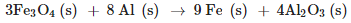Solution:

In the above reaction oxygen is transferred from Fe3O4 to Al. so Fe3O4 is reduced while Al is oxidised.

QUESTION: 12

Fractional Oxidation Number exists because

Solution:

Fractional Oxidation Number exists because the element is present in different oxidation states.

QUESTION: 13

For ions composed of only one atom, the oxidation number is equal to the

Solution:

For ions the oxidation state is equal to the charge of ion.

QUESTION: 14

Which of the following elements does not show disproportionation tendency?

Solution:

F is most electronegative element so it always show -1 oxidation state.

QUESTION: 15

The oxidation state of iron in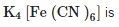Solution:

CN carries -1 charge so oxidation state of Fe will be +2.

QUESTION: 16

The formation of sodium chloride involves two half reaction, which clearly show involvement of electrons. 2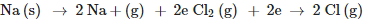Sum of the half reactions gives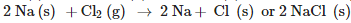In terms of electron-transfer change, name the oxidising agent(s) and reducing agent(s)

Solution:

oxidizing agent is one which itself get reduced and make the other substance oxidized. While reducing

QUESTION: 17

The strength of a reductant/oxidant in redox systems can be determined

Solution:

The reductant/oxidant in redox system can be determined by titration method.

QUESTION: 18

Hydrogen is prepared from H2O by adding

Solution:

Ca is added which act as a reducing agent.

QUESTION: 19

Consider the elements: Cs, Ne, I and F. Identify the element(s) that exhibits only Positive oxidation state

Solution:

Cs is alkali metal so will show positive oxidation state of +1

QUESTION: 20

The standard emf of a galvanic cell involving 3 moles of electrons in its redox reaction is 0.59 V. The equilibrium constant for the reaction of the cell is ______.

Solution: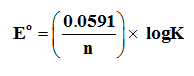QUESTION: 21

Redox reaction between copper and aqueous solution of silver nitrate happens when copper rod is dipped in silver nitrate solution. What happens in the final stage?

Solution: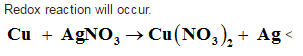QUESTION: 22

One of the following has application in electrode processes and cells

Solution:

The concept of redox reaction is used.

QUESTION: 23

An oxidation number of +1 is found in all their compounds of one of the below given options

Solution:

Alkali metals have +1 oxidation state.

QUESTION: 24

The exhibition of various oxidation states by an element is also related to the outer orbital electronic configuration of its atom. Atom(s) having which of the following outermost electronic configurations will exhibit more than one oxidation state in its compounds.

Solution:

(A) is the configuration of transition element which shows variable oxidation state.

QUESTION: 25

Zn2+/Zn represents

Solution:

This is redox couple.